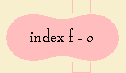# Lissajous curve

## higher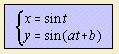where 0 < b < π/2.

When the constant a is rational, the curve is algebraic and  closed.
If a is irrational, the curve fills the area [-1,1] x [-1,1].
It is easy to see that the curves for 1/a and a are equal in form. This means that we can confine ourselves to the case a < 1.

Jules-Antoine Lissajous (1822-1880) discovered these elegant curves (in 1857) while doing his sound experiments.
But it is said that the American Nathaniel Bowditch (1773-1838) found the curves already in 1815. After him the curve bears the name of Bowditch curve.
Another name that I found is the play curve of Alice1.

The curves are constructed as a combination of two perpendicular harmonic oscillations. Patterns occur as a result of differences in frequency ratio (a) and phase (b).
At high school we used the oscilloscope to make the curve visible (nowadays a computer would do), by connecting different harmonic signals to the x- and y-axis entrance.

The curves have applications in physics, astronomy and other sciences.

Each Lissajous curve can be described with an algebraic equation.

Write a as the smallest integers m, n for which a = m/n. Then the degree of the equation obeys the following rules:

• degree = m
for b = 0 and m is odd
for b = π/2 and m is even
• degree = 2m
for b ∈ ]0,π/2] and m is odd
for b ∈ [0,π/2[ and m is even

Some examples of Lissajous curves are the following:
 a b name of the curve 1 0 straight line 1 π/2 circle 1 <> 0, π/2 ellipse 2 π/2 parabola 2 <> π/2 besace: its parameter a is equal to the tangent of the Lissajous curve's parameter b. 3 0 the cubic y = 2x3 - x (see the picture below) 3 π/2 the sextic y2 = (1 - x2)(1-4x2)2 (see the picture below)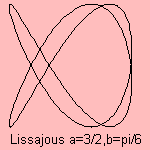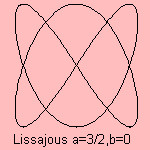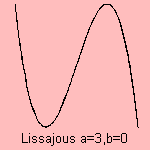In the situation that the parameter a is an integer, three forms of the curve can be distinguished:

1. the curve is symmetric with respect to the x- and y-axis
When a is even: b = 0. When a is odd: b = π/2.
2. the curve is asymmetric
For this form (and the one before, as a matter of fact) the number of compartments is equal to the value of a.
3. the curve has no compartments, the two parts of the curve come together
When a is even: b = π/2. When a is odd: b = 0.

There is a relation with the Tchebyscheff polynomial: the Lissajous curve (a, b) corresponds with Tchebyscheff function Ta for a integer and b=π/2 (for even a) or b=0 (for odd a).

The Lissajous curve can be extended to the

notes

1) In French: joue courbe d'Alice.